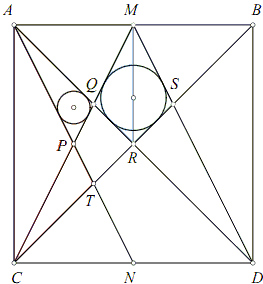# Proportions in Square

A 1835 Sangaku from the Miyagi prefecture {Fukagawa & Pedoe, 3.1.5] reached us with no solution. Two solutions have been posted by J. Marshall Unger from Ohio State University in his write-up of sangaku problems, (Problem #23).

Given square ABCD, with M, N the midpoints of AB, CD, inscribe a circle in kite QRSM and in triangle APQ.

Prove that the radius of the smaller circle is half that of the larger circle.I find both solutions enticingly elegant and sufficiently simple to deserve inclusion in my collection. The problem also links to a separate problem of dividing a segment into equal parts which served as an extra inducement for putting up the current page.

Solutions

### References

1. H. Fukagawa, D. Pedoe, Japanese Temple Geometry Problems, The Charles Babbage Research Center, Winnipeg, 1989.

Write to:

Charles Babbage Research Center
P.O. Box 272, St. Norbert Postal Station
Winnipeg, MB

## SangakuGiven square ABCD, with M, N the midpoints of AB, CD, inscribe a circle in kite QRSM and in triangle APQ.

Prove that the radius of the smaller circle is half that of the larger circle.### Solution 1

Observe that, for any inscriptible shape, the inradius could be found as the ratio of the area and the semiperimeter. We shall juxtapose ΔAQP and the kite MQRS.

Point P is the intersection of the diagonals in rectangle ACNM; hence P is exactly as far from AM as R which is the center of the square ABCD. It follows that triangles AMP and AMR have the same area and so are triangles AQP and MQR obtained from the former by removing the common part - ΔAMQ. We conclude that the area of the kite MQRS is twice that of ΔAQP.

Triangles MQR and CQA are similar and, since 2·MR = AC,

MQ + QR + RS + MS = 2(MQ + QR) = CQ + AQ.

But, by symmetry, CQ = AT = AP + PT, while clearly PT = PQ. Therefore,

CQ + AQ = AT + AQ = AP + PQ + AQ.

A combination of the two identities shows that ΔAQP and the kite MQRS have equal perimeters and, hence, semiperimeters, thus proving the claim.

### Solution 2

Note that the incircle of the kite is also the incircle of ΔDQM. Further, AN||DM, implying similarity of triangles DQM and AQP. Since DM is twice AP, the triangles are similar with the coefficient of 2, so that their inradii are in the same proportion.

### Remark

This is a well known fact (that arises in the problem of division of a segment into equal parts) that 2·AQ = QR. Thus also 2·MQ = CQ. Now, since CP = MP, 2·PQ = QM. This remark could be employed in both of the solutions above.